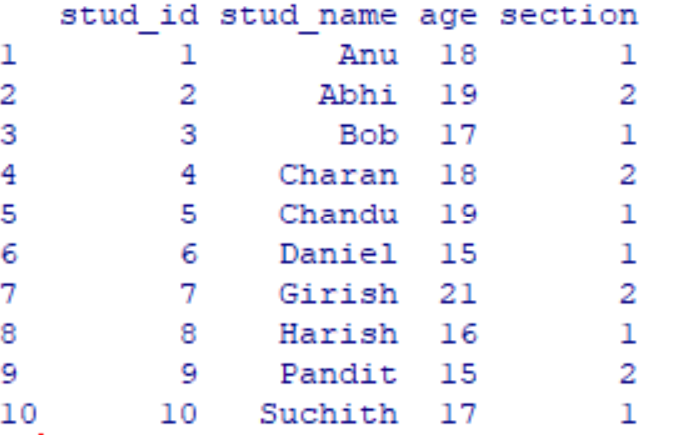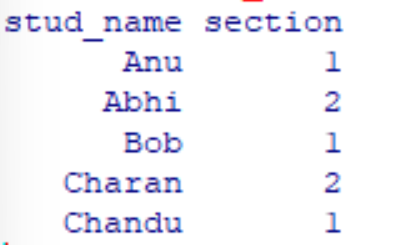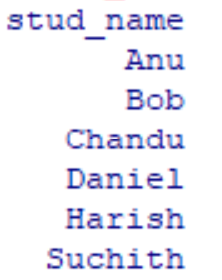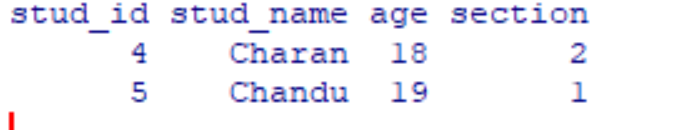Open in App
Not now

# How to select a subset of DataFrame in R

• Difficulty Level : Medium
• Last Updated : 12 Jul, 2022

In general, when we were working on larger dataframes, we will be only interested in a small portion of it for analyzing it instead of considering all the rows and columns present in the dataframe.

Creation of Sample Dataset

Let’s create a sample dataframe of Students as follows

## R

 `student_details < -``data.frame``(` `    ``stud_id=``c``(1: 10),` `    ``stud_name=``c``(``"Anu"``, ``"Abhi"``, ``"Bob"``, ` `                ``"Charan"``, ``"Chandu"``,` `                ``"Daniel"``, ``"Girish"``, ``"Harish"``,` `                ``"Pandit"``, ``"Suchith"``),` `    ``age=``c``(18, 19, 17, 18, 19, 15, 21,` `          ``16, 15, 17),` `    ``section=``c``(1, 2, 1, 2, 1, 1, 2, 1,` `              ``2, 1)` `)` `print``(student_details)`

Output:## Method 1. Using Index Slicing

This method is used when the analyst was aware of the row/ column numbers to extract from the main dataset and create a subset from them for easy analysis. The numbers given to those rows or columns are called Index(s).

Syntax: dataframe[rows,columns]

Example: To make a subset of the dataframe of the first five rows and the second and fourth column

## R

 `subset_1<-student_details[``c``(1:5),``c``(2,4)]` `print``(subset_1)`

Output:## Method 2. Using subset() function

When the analyst is aware of row names and column names then subset() method is used. Simply, This function is used when we want to derive a subset of a dataframe based on implanting some conditions on rows and columns of the dataframe. This method is more efficient and easy to use than the Index method.

Syntax: subset(dataframe,rows_condition,column_condition)

Example: Extract names of students belonging to section1

## R

 `subset_2=``subset``(student_details,section==1,stud_name)` `print``(subset_2)`

Output:## Method 3. Using dplyr package functions

In the filter()- this function is used when we want to derive a subset of the dataframe based on a specific condition.

This method is used when analysts want to derive a subset based on some condition either on rows or columns or both using row and column names. Among above mentioned three methods this method is efficient than the other two.

Syntax: filter(dataframe,condition)

Note: Make sure you installed dplyr package in the Workspace Environment using commands

```install.packages("dplyr") -To install

Example: Let’s extract rows that contain student names starting with the letter C.

## R

 `library``(dplyr)` `subset_3 < -``filter``(student_details,` `                   ``startsWith``(stud_name, ``'C'``))` `print``(subset_3)`

Output:My Personal Notes arrow_drop_up
Related Articles